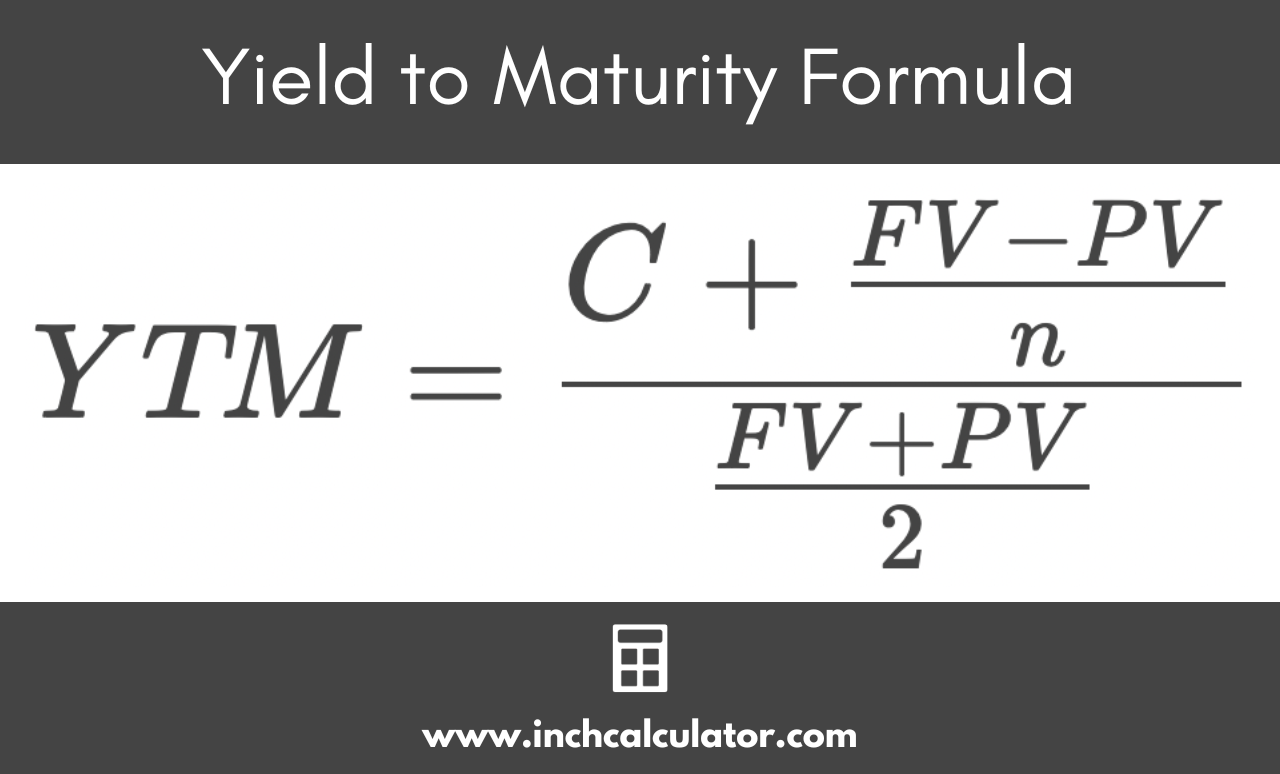# Yield to Maturity Calculator – Calculate YTM for Bonds

Use our YTM calculator to find the yield to maturity for a bond by entering the information about the bond below.


%

## Results:

 Yield to Maturity: % Current Yield: %
Learn how we calculated this below

## How to Calculate Yield to Maturity

Calculating the yield to maturity is essential for anyone who wants to compare bonds and decide whether a particular bond they are considering will be a good investment.

When all else is equal, bonds with higher yields to maturity are a better investment than those with lower yields to maturity.

The yield to maturity—which is effectively an annualized rate of return—represents the level of return a bondholder can expect to receive. The yield to maturity and bond price are directly related to one another.

Understanding how to calculate yield to maturity will make it much easier for prospective investors to compare different types of bonds, such as coupon bonds versus zero-coupon bonds and bonds that have different lengths of time before maturity.

This brief guide discusses how to calculate yield to maturity with examples.

### What is Yield to Maturity?

Yield to Maturity (YTM) represents the total return that is generated once a bond has paid all coupon payments and reached maturity, which is when the face value of the bond will be paid to the bondholder.

In other words, YTM represents an annualized figure of what bondholders can expect to receive. Bonds, unlike stocks, do not offer speculative paybacks. The coupon payments and future value have already been determined and the only way for the bond issuer to not pay them is to default.

YTM calculations typically include a few basic assumptions. Namely, that the bond will be paid as promised (i.e., there is no default) and the bond will be held until maturity. YTM is also sometimes referred to as a bond’s redemption yield.

### Yield to Maturity FormulaIn order to calculate the YTM for a coupon-issuing bond, you must know the coupon rate, the bond’s face value, the present value (which should equal the current price), and the number of years to maturity.

With most bonds, this information should be clearly evident on the bond itself. But if you don’t have the physical bond in front of you, you can easily find this information within any bond-holding account.

You can use the following formula to calculate a bond’s yield to maturity:

YTM = \frac{ C + \frac{FV − PV}{n} }{ \frac{FV + PV}{2} }

Where:
C = coupon rate
FV = face value
PV = present value (current price)
n = years to maturity

To make the process simpler, you can also use a bond yield calculator.

### Example

Suppose you are considering a bond that has a face value of $1,100, a current price of$1,000, an annual coupon rate of 5 percent, and a time to maturity of 10 years. This bond pays its coupons annually.

To calculate the YTM, all you need to do is use the YTM formula above. In this example:

YTM = \frac{ \$55 + \frac{\$1,100 − \$1,000}{10} }{ \frac{\$1,100 + \$1,000}{2} } YTM = \frac{ \$55 + \frac{\$100}{10} }{ \frac{\$2,100}{2} }
YTM = \frac{ \$55 + \$10 }{ \$1,050 } YTM = 6.19\% The estimated YTM is 6.19%, but the exact YTM is 6.25 percent, which is a bit higher. This is because the formula above provides a reasonable estimate, but the exact yield to maturity requires a more complex mathematical equation to solve. ## Yield to Maturity Formula for Zero-Coupon Bonds Not all bonds pay coupons every period. Zero-coupon bonds pay the entire payout only once the bond has fully matured. In order to calculate the YTM, you can use a bond yield calculator or do the calculations by hand. To calculate using the formula below, you will need the bond’s face value, the present value (or the current price), and the number of years to maturity. ### Zero-Coupon (Discount) Bond YTM Formula You can use this formula to calculate the YTM for zero-coupon bonds: YTM = \sqrt[n]{ \frac{FV}{PV} } − 1 Where: FV = face value PV = present value (current price) n = years to maturity Additionally, you could use the formula for coupon-yielding bonds and simply enter the coupon rate as zero. ### Example In this example, we will use a zero-coupon bond with the same variables as the last example—its time to maturity is 10 years, the present value of the bond is$1,000, and the future value of the bond is $1,100. Simply plug the numbers in: YTM = \sqrt{ \frac{\$1,100}{\\$1,000} } − 1
YTM = 0.9577\%

As you can see, the yield to maturity is much worse for the zero-coupon bond compared to its coupon-paying counterpart because the coupons themselves are a primary driver of value.

You might also be interested in our stock profit calculator.